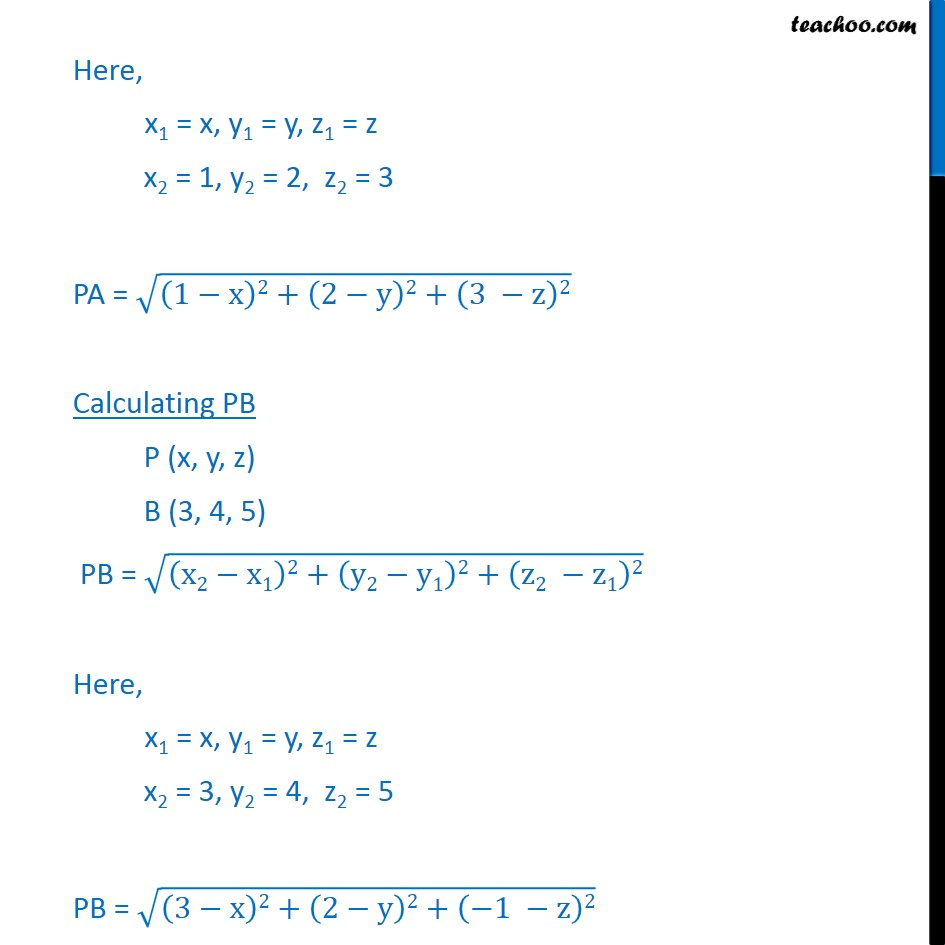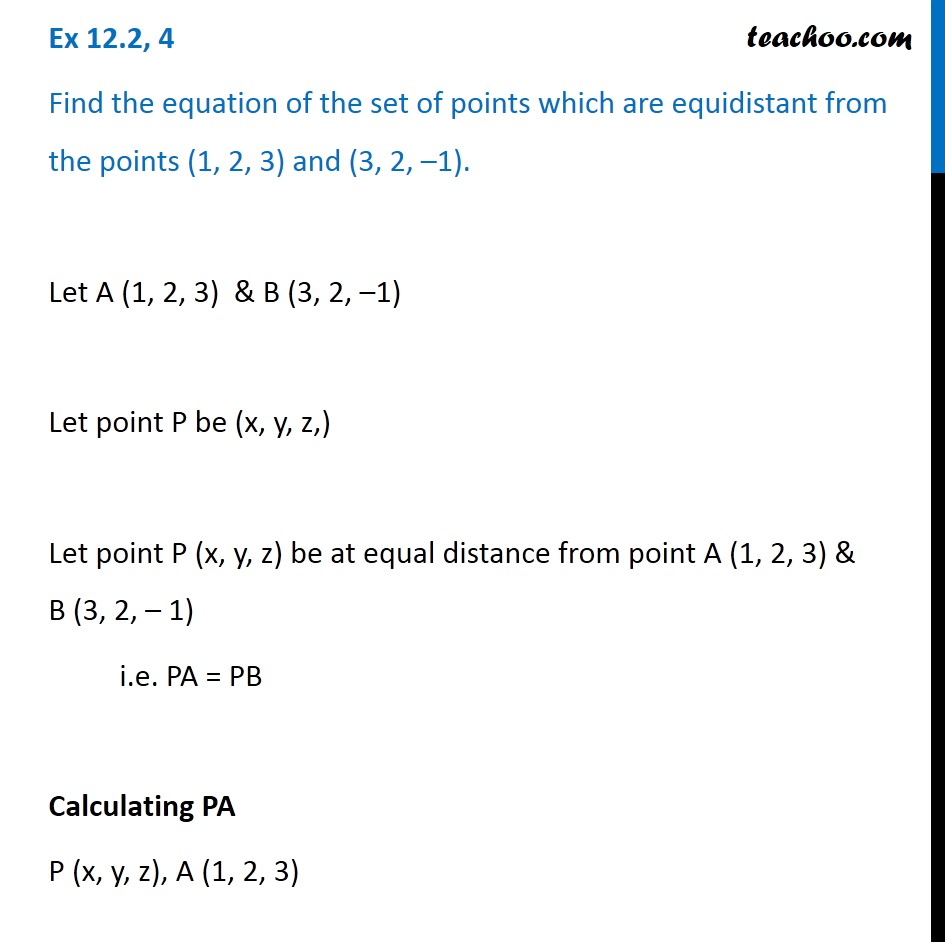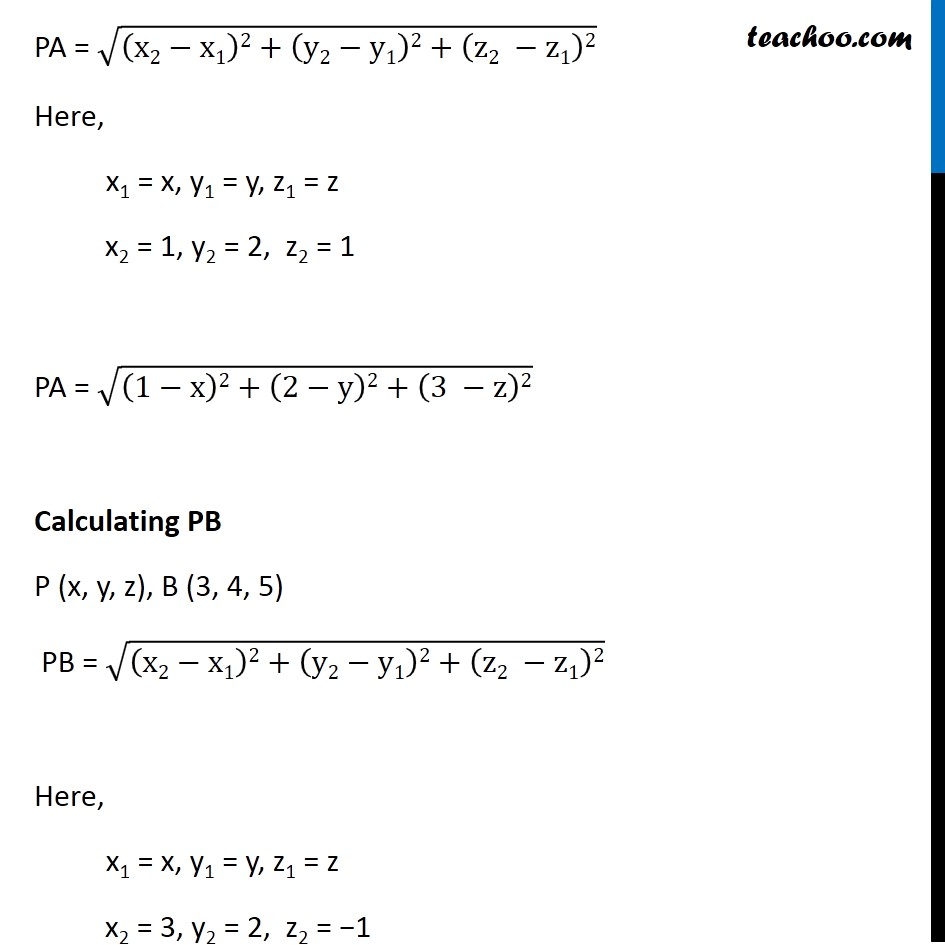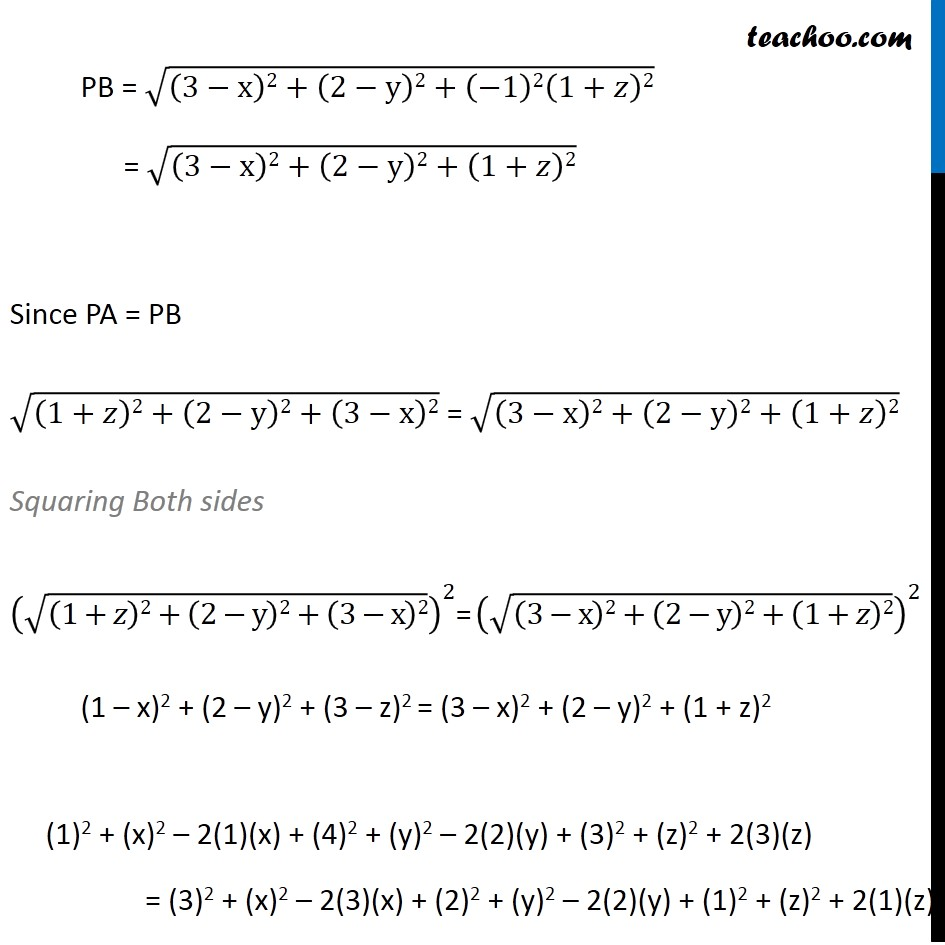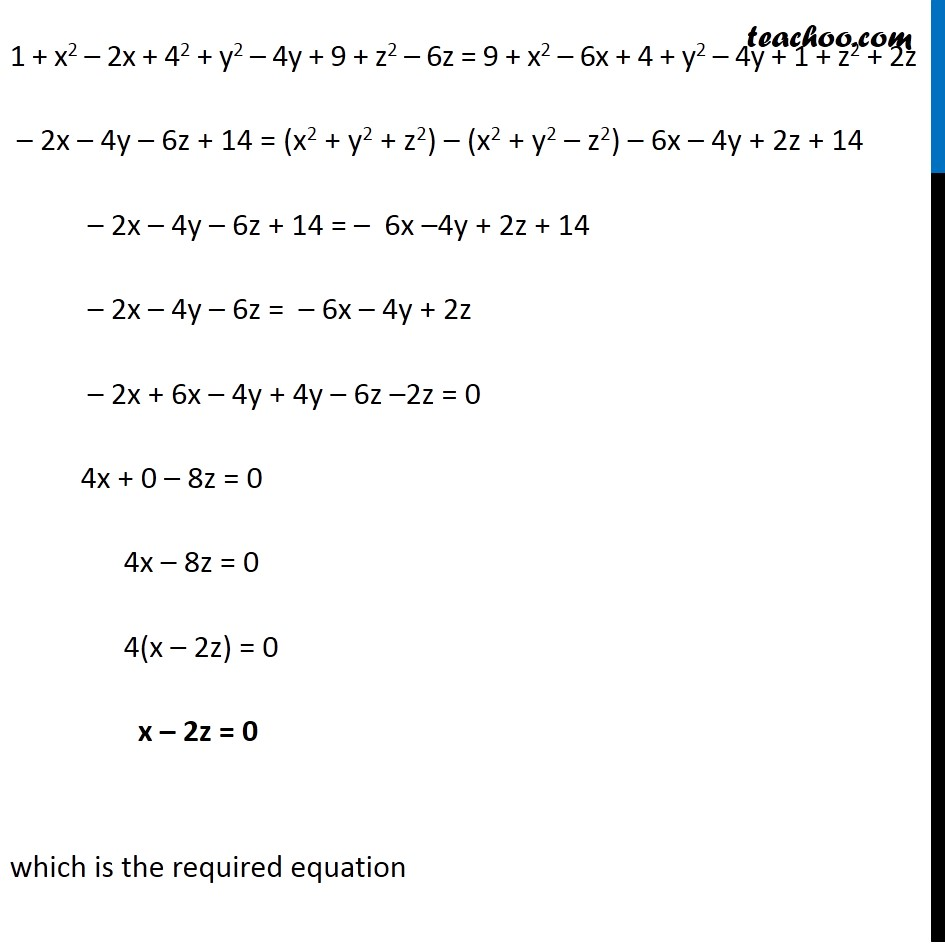1. Chapter 12 Class 11 - Intro to Three Dimensional Geometry (Term 2)
2. Concept wise
3. Distance between two points - Set of points

Transcript

Ex 12.2, 4 Find the equation of the set of points which are equidistant from the points (1, 2, 3) and (3, 2, –1). Let A (1, 2, 3) & B (3, 2, –1) Let point P be (x, y, z,) Let point P (x, y, z) be at equal distance from point A (1, 2, 3) & B (3, 2, – 1) i.e. PA = PB Calculating PA P (x, y, z), A (1, 2, 3) PA = √((x2−x1)2+(y2−y1)2+(z2 −z1)2) Here, x1 = x, y1 = y, z1 = z x2 = 1, y2 = 2, z2 = 1 PA = √((1−x)2+(2−y)2+(3 −z)2) Calculating PB P (x, y, z), B (3, 4, 5) PB = √((x2−x1)2+(y2−y1)2+(z2 −z1)2) Here, x1 = x, y1 = y, z1 = z x2 = 3, y2 = 2, z2 = −1 PB = √((3−x)2+(2−y)2+(−1 −z)2) PB = √((3−x)2+(2−y)2+(−1)2(1+𝑧)2) = √((3−x)2+(2−y)2+(1+𝑧)2) Since PA = PB √((1+𝑧)2+(2−y)2+(3−x)2) = √((3−x)2+(2−y)2+(1+𝑧)2) Squaring Both sides (√((1+𝑧)2+(2−y)2+(3−x)2))^2= (√((3−x)2+(2−y)2+(1+𝑧)2))^2 (1 – x)2 + (2 – y)2 + (3 – z)2 = (3 – x)2 + (2 – y)2 + (1 + z)2 (1)2 + (x)2 – 2(1)(x) + (4)2 + (y)2 – 2(2)(y) + (3)2 + (z)2 + 2(3)(z) = (3)2 + (x)2 – 2(3)(x) + (2)2 + (y)2 – 2(2)(y) + (1)2 + (z)2 + 2(1)(z) 1 + x2 – 2x + 42 + y2 – 4y + 9 + z2 – 6z = 9 + x2 – 6x + 4 + y2 – 4y + 1 + z2 + 2z – 2x – 4y – 6z + 14 = (x2 + y2 + z2) – (x2 + y2 – z2) – 6x – 4y + 2z + 14 – 2x – 4y – 6z + 14 = – 6x –4y + 2z + 14 – 2x – 4y – 6z = – 6x – 4y + 2z – 2x + 6x – 4y + 4y – 6z –2z = 0 4x + 0 – 8z = 0 4x – 8z = 0 4(x – 2z) = 0 x – 2z = 0 which is the required equation